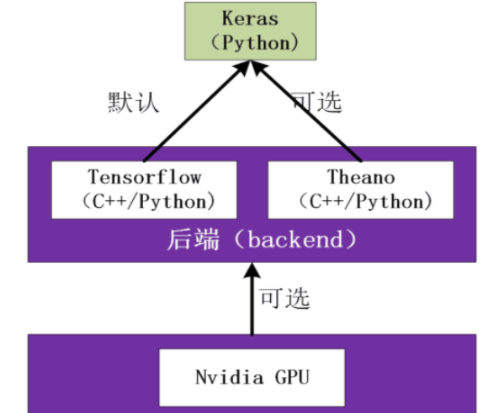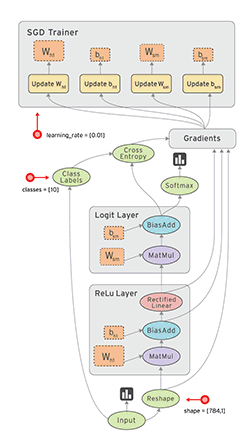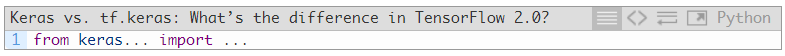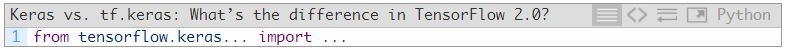# 【深度学习】Keras和Tensorflow框架使用区别辨析1人已赏
Fizz 发布于 2021-05-17 17:58:58 浏览 2770 点赞 94 收藏 7

## 【深度学习】Keras和Tensorflow框架使用区别辨析

``````文章目录
1 概述
2 Keras简介
3 Tensorflow简介
4 使用tensorflow的几个小例子
5 Keras搭建CNN
6 tensorflow和keras版本对应关系
7 TensorFlow 2.0中的tf.keras和Keras有何区别？为什么以后一定要用tf.keras？``````

# 1 概述

Keras 已经被大规模采用，并与 TensorFlow 集成在一起。这种 TensorFlow + Keras 的组合让你可以：

# 2 Keras简介

Keras是基于TensorFlow和Theano（由加拿大蒙特利尔大学开发的机器学习框架）的深度学习库，是由纯python编写而成的高层神经网络API，也仅支持python开发。它是为了支持快速实践而对tensorflow或者Theano的再次封装，让我们可以不用关注过多的底层细节，能够把想法快速转换为结果。它也很灵活，且比较容易学。Keras默认的后端为tensorflow，如果想要使用theano可以自行更改。tensorflow和theano都可以使用GPU进行硬件加速，往往可以比CPU运算快很多倍。因此如果你的显卡支持cuda的话，建议尽可能利用cuda加速模型训练。（当机器上有可用的GPU时，代码会自动调用GPU 进行并行计算。） 目前Keras已经被TensorFlow收录，添加到TensorFlow 中，成为其默认的框架，成为TensorFlow官方的高级API。CUDA GPU加速。

CUDA 是NVIDIA推出的用于自家 GPU 的并行计算框架，也就是说 CUDA 只能在NVIDIA的 GPU 上运行，而且只有当要解决的计算问题是可以大量并行计算的时候才能发挥 CUDA 的作用。

# 3 Tensorflow简介

tensorflow是Google开源的基于数据流图的深度学习框架，支持python和c++程序开发语言。轰动一时的AlphaGo就是使用tensorflow进行训练的，其命名基于工作原理，tensor 意为张量（即多维数组），flow 意为流动。即多维数组从数据流图一端流动到另一端。目前该框架支持 Windows、Linux、Mac乃至移动手机端等多种平台。下图为其数据流动示意图。# 4 使用tensorflow的几个小例子

``````import tensorflow as tf

import os
os.environ["TF_CPP_MIN_LOG_LEVEL"]= "3"

a = tf.constant(5,name = 'input_a')
b = tf.constant(3,name = 'input_b')
c = tf.multiply(a,b,name='mul_c')
sess = tf.Session()
print(sess.run(a))
print(sess.run(b))
print(sess.run(c))
print(sess.run(d))
print(sess.run(e))
``````
``````import tensorflow as tf

import os
os.environ["TF_CPP_MIN_LOG_LEVEL"]= "3"

a = tf.constant([5,3],name = 'input_a')
b = tf.reduce_prod(a,name = 'pro_b')
c = tf.reduce_sum(a,name = 'sum_c')

sess = tf.Session()
print(sess.run(a))
print(sess.run(b))
print(sess.run(c))
print(sess.run(d))
``````
``````import tensorflow as tf

import os
os.environ["TF_CPP_MIN_LOG_LEVEL"]= "3"

#一行两列的张量
m1 = tf.constant([[2,2]])
#两行一列的张量
m2 = tf.constant([,])
dot_operation = tf.matmul(m1,m2)

#方法一
sess = tf.Session()
result = sess.run(dot_operation)
print(result)

#方法二
with tf.Session() as sess:
result = sess.run(dot_operation)
print(result)
``````
``````import tensorflow as tf

import os
os.environ["TF_CPP_MIN_LOG_LEVEL"]= "3"
#创建未知的张量
x1 = tf.placeholder(dtype=tf.float32,shape=None)
y1 = tf.placeholder(dtype=tf.float32,shape=None)
z1 = x1 + y1

x2 = tf.placeholder(dtype=tf.float32,shape=[2,1])
y2 = tf.placeholder(dtype=tf.float32,shape=[1,2])
z2 = tf.matmul(x2,y2)

with tf.Session() as sess:
#一个一个处理
#z1_value = sess.run(z1,feed_dict={x1:1,y1:2})

#一起处理
z1_value,z2_value = sess.run([z1,z2],feed_dict={
x1:1,y1:2,x2:[,],y2:[[3,3]]
})
print(z1_value)
print(z2_value)
``````

3.0 [[6. 6.] [6. 6.]]

``````#函数y= 0.1x + 0.3
import tensorflow as tf
#numpy是一个有关科学计算的模块
import numpy as np

#防止他输出一些没用的东西
import os
os.environ["TF_CPP_MIN_LOG_LEVEL"]= "3"

#创造数据,用这些数据去训练神经网络
x_data = np.random.rand(100).astype(np.float32)
y_data = x_data*0.1 + 0.3

#开始创建结构
Weights = tf.Variable(tf.random_uniform(,-1.0,1.0))
biases = tf.Variable(tf.zeros())

y = Weights*x_data + biases

loss = tf.reduce_mean(tf.square(y-y_data))
train = optimizer.minimize(loss)

init = tf.global_variables_initializer()
#-----------------------------------------------
sess = tf.Session()
sess.run(init)

for step in range(201):
sess.run(train)
if step%20 == 0:
print(step,sess.run(Weights),sess.run(biases))
``````

# 5 Keras搭建CNN

``from keras.layers import Dense, Activation, Convolution2D, MaxPooling2D, Flatten``

``````model.add(Convolution2D(

batch_input_shape=(64, 1, 28, 28),

filters=32,

kernel_size=5,

strides=1,

data_format='channels_first',

))

,

``````model.add(MaxPooling2D(

pool_size=2,

strides=2,

data_format='channels_first',

))``````

``````model.add(Convolution2D(64, 5, strides=1, padding='same', data_format='channels_first'))

``````model.add(Flatten())

``````model.add(Dense(10))

``````model.compile(optimizer=adam,
loss='categorical_crossentropy',
metrics=['accuracy'])

loss='categorical_crossentropy',

metrics=['accuracy'])``````

``model.fit(X_train, y_train, epoch=1, batch_size=32,)``

# 6 tensorflow和keras版本对应关系

Tensorflow版本 Keras版本 Tensorflow 2.1 Tensorflow 2.0 Tensorflow 1.15 Keras 2.3.1

Tensorflow 1.14 Keras 2.2.5

Tensorflow 1.13 Tensorflow 1.12 Tensorflow 1.11 Keras 2.2.4

Tensorflow 1.10 Tensorflow 1.9 Keras 2.2.0

Tensorflow 1.8 Tensorflow 1.7 Tensorflow 1.5 Keras 2.1.6

Tensorflow 1.4 Keras 2.0.8

Tensorflow 1.3 Tensorflow 1.2 Tensorflow 1.1 Tensorflow 1.0 Keras 2.0.6

Tensorflow 0.12 Keras 1.2.2

# 7 TensorFlow 2.0中的tf.keras和Keras有何区别？为什么以后一定要用tf.keras？

Keras v2.3.0 是 Keras 第一个与 tf.keras 同步的版本，也将是最后一个支持除 TensorFlow 以外的后端（即 Theano，CNTK 等）的主要版本。最重要的是，深度学习从业人员应该开始使用 TensorFlow 2.0 和 tf.keras 软件包了。*本文仅代表作者观点，不代表易百纳技术社区立场。系作者授权易百纳技术社区发表，未经许可不得转载。

### 相关专栏海思讨论群君正智能视频群客服微信： x460910098客服手机： 17714209983